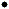Ivermectin Paste
DEFINITION
Ivermectin Paste contains NLT90.0%(RB 1-Jul-2011) and NMT 110.0% of the labeled amount of Ivermectin, calculated as the sum of component H2B1a (C48H74O14) and component H2B1b (C47H72O14). The ratio of the contents, H2B1a/(H2B1a + H2B1b), is NLT 90.0%.
IDENTIFICATION
Sample solution:  0.5 mg/mL of ivermectin dispersed in methanol from a quantity of Paste. Sonicate if necessary until completely dispersed.
Application volume:  2 µL
Developing solvent system:  Methylene chloride, methanol, and ammonium hydroxide (90:9:1)
Analysis:  Develop the chromatogram in an unsaturated chamber. Remove the plate, allow to air dry, and examine under short- and long-wavelength UV light.
Acceptance criteria:  Meets the requirements
•  B. The retention times of the two principal component peaks of ivermectin from the Sample solution correspond to those of the two principal component peaks of ivermectin from the Standard solution, as obtained in the Assay.
ASSAY
•  Procedure
Mobile phase:  Acetonitrile, methanol, and water (106:55:39)
Standard solution:  0.4 mg/mL of USP Ivermectin RS in methanol
Sample solution:  Disperse a quantity of Paste in methanol, using sonication if necessary, to obtain a solution containing 0.4 mg/mL of ivermectin.
Chromatographic system
Mode:  LC
Detector:  UV 245 nm
Column:  4.6-mm × 25-cm; 5-µm packing L1
Flow rate:  1.5 mL/min
Injection size:  20 µL
System suitability
Sample:  Standard solution
Suitability requirements
Resolution:  NLT 3.0 between the first peak (component H2B1b) and the second peak (component H2B1a)
Relative standard deviation:  NMT 2.0%, determined from the component H2B1a peak
Analysis
Samples:  Standard solution and Sample solution
Calculate the percentage of the labeled amount of ivermectin, component H2B1a (C48H74O14) and component H2B1b (C47H72O14), in the portion of Paste taken:
Result = (rU/rS) × (CS/CU) × 100
 rU = = sum of the peak responses for component H2B1a and component H2B1b from the Sample solution rS = = sum of the peak responses for component H2B1a and component H2B1b from the Standard solution CS = = concentration of USP Ivermectin RS in the Standard solution (mg/mL) CU = = nominal concentration of ivermectin in the Sample solution (mg/mL)
Calculate the ratio of the contents, in percentage, of the components, H2B1a/(H2B1a + H2B1b), in the portion of Paste taken:
Result = (rU/rT) × 100
 rU = = peak response of H2B1a from the Sample solution rT = = sum of the peak responses for component H2B1a and component H2B1b from the Sample solution
Acceptance criteria:90.0%(RB 1-Jul-2011)–110.0% of the labeled amount of ivermectin, calculated as the sum of component H2B1a (C48H74O14) and component H2B1b (C47H72O14). The ratio of the contents, H2B1a/(H2B1a + H2B1b), is NLT 90.0%.
IMPURITIES
•  Organic Impurities
Mobile phase, Sample solution, and Chromatographic system:  Proceed as directed in the Assay.
Standard solution:  0.004 mg/mL of USP Ivermectin RS in methanol
Analysis
Samples:  Standard solution and Sample solution
Calculate the percentage of each impurity in the portion of Paste taken, disregarding any peak below 0.05%:
Result = (rU/rS) × (CS/CU) × 100
 rU = = peak response for each impurity from the Sample solution rS = =peak response of the principal peak(RB 1-Jul-2011) from the Standard solution CS = = concentration of the Standard solution (mg/mL) CU = = nominal concentration of ivermectin in the Sample solution (mg/mL)
Acceptance criteria:  NMT3.0%(RB 1-Jul-2011) ofany(RB 1-Jul-2011) peak with a relative retention time of 1.3–1.5, relative to that of the principal peak
Any other impurity:  NMT 1.0%
Total impurities:  NMT 6.0%
•  Packaging and Storage: Preserve in well-closed containers. Store at a temperature not higher than 30.